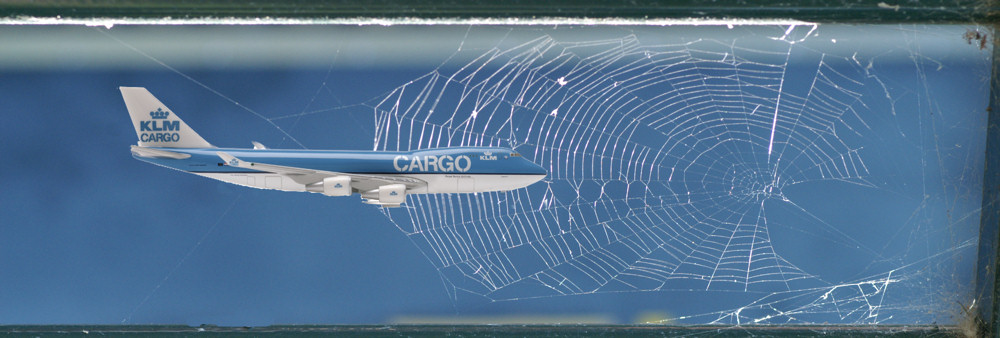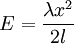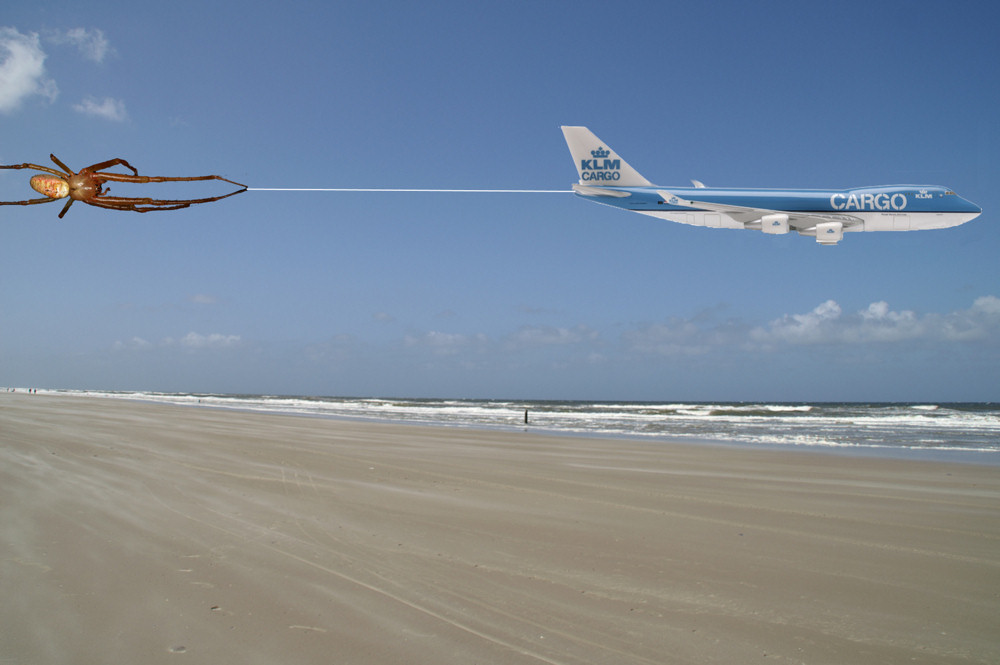﻿ How thick should a spider silk thread be to stop a Boeing-747 in full flight?

# How thick should a spider silk thread be to stop a Boeing-747 in full flight?

Back <------"A pencil thick spider's silk thread is capable of stopping a Boeing-747 in full flight. "
Ten years ago I found this statement somewhere in a book and placed this statement on my site without controlling the sources. So now and then people write me to ask where I found this statement and if the Boeing could be stopped with this pencil thick line. Now in 2007 it is much easier to check these statements on the internet and the first check was in www.books.google.com . I has already tried to retrieve the statement from my own books at home but failed.
Google did found some references but all the books where published after 1996 and it was almost certain the phrases were copied from this site because the sentence were almost identical.

The strength of a thread depends on more than one feature. One can hang a weight on a given thread and measure the weight before it breaks. But also the elasticity of a thread is part of its strength. Also its weight per volume is an feature that is added to its strength. The less weight per volume, the better. We will discard this last feature in our calculations for simplification.

The data:

A 747-400 typically takes off at 180 mph (290 km/h), cruises at 565 mph (910 km/h) and lands at 160 mph (260 km/h).
Typical operating empty weight is 180,000 kg.
Sullivan cited that the breaking stress of the dragline silk for three species of spiders range from 1420 to 1550 million N/m2 with elongation at break ranging from 16 to 30%. The density of this silk is 1300 kg/m3.
In our calculations we will use a breaking stress of 1500 million N/m2 and 30% break elongation and a plane of 60000 kg flying 1080 km/h = 300 m/s.
When we use a thread with a length of 1000 meter the plane must be stopped in 300 meters.
We assume the thread is weightless and is tough enough to stop the plane when stretched completely.
We also assume that the silk thread follows Hooke's law of elasticity that states that the amount by which a material body is deformed (the strain) is linearly related to the force causing the deformation (the stress). Stress and strain are assumed linear.
We will also assume that all kinetic energy of the moving plane will be changed to the potential energy that will be stored in the thread.
The kinetic energy will be calculated with the formula: E (J) = 0.5 m (N) * v ^2 (m^2/s^2) and the elastic potential energy with the formula:

Intermezzo -------------------------------------------------
The elastic potential energy is defined as a work needed to compress or expand an elastic body.
The potential energy of a string or spring that has modulus of elasticity under an extension of x is thenThis formula is obtained from the integral of Hooke's law:Elastic Potential Energy is the kind of energy that is stored in a bow, or in a spring or a silken thread that stops a Boeing-747
The energy stored = the work done to stretch the bow, so:
Elastic Energy (joules) = Average Force (newton) x Distance (meters)
End intermezzo -------------------------------------------

Elastic Energy (joules = Nm) = 0.5 K (N) x Distance (m)
Diameter = K (N) / breaking stress (N/m2)
Surface = pi / 4 * diameter^2

Now the question raises: How many spiders do we need to make such a thread? We made two calculations. One for a small Araneus diadematus thread of 0.5 um and one for a thick Nephila thread of 10 um. For the calculation of the surface of the thread was assumed square also for simplification.
Réaumur calculated that 27648 female spiders (Araneus diadematus) would be required to produce 1 lb of silk. In the calculation this is compared with the amount of Nephila spiders needed with 10 um thick silk lines. These results are in good agreement and not with the amount of Araneus diadematus spiders! If one uses 11.25 um thickness for a thread the results equal.
We assumed that one spider can give us a thread of 100 meters.
In the last calculations we wondered how much the temperature would rise if all the energy is transformed into heat. We used for the silk the specific heat capacity of water of 1 J/gK.

The calculations:

 Thread length l 1000 m 1000 m 30000 m Initial velocity u 80 m/s 300 m/s 80 m/s stretch % 30% 30% 30% weight plane m 180000 Kg 180000 Kg 180000 Kg distance to stop s 300 m 300 m 9000 m Breaking force K 1.50E+09 N/m2 1.50E+09 N/m2 1.50E+09 N/m2 accelaration a -10.67 m/s2 -150.00 m/s2 -0.36 m/s2 E=0.5mv2 E 576000000 Nm 8.1E+09 Nm 576000000 Nm K=E/(0.5s) K 3840000 N 54000000 N 128000 N Surface thread d 2.56E-03 m2 3.60E-02 m2 8.53E-05 m2 Diameter thread to stop Boeing-747 5.7 cm 21.4 cm 1.0 cm No of spiders needed on basis of diameter and 10 spiders needed for one km of thread diameter thread um 0.5 10 0.5 10 0.5 10 surface thread m2 2.5E-13 1E-10 2.5E-13 1E-10 2.5E-13 1E-10 No of threads 1.02E+10 2.56E+07 1.44E+11 3.60E+08 3.41E+08 8.53E+05 No of spiders (10/km) 1.02E+11 2.56E+08 1.44E+12 3.60E+09 1.02E+11 2.56E+08 No of billion spiders 102 1440 102 Number of Nephila spiders needed when assumed 27648 spider produce 1 lb silk Number of spiders / kg 60952 n/kg 60952 n/kg 60952 n/kg density silk 1300 kg/m3 1300 kg/m3 1300 kg/m3 volume silk 2.56 m3 36 m3 2.56 m3 kg silk 3328 kg 46800 kg 3328 kg Number of spiders Nephila 2.03E+08 2.85E+09 2.03E+08 Number of billion spiders Nephila 0.20 0.26 2.85 3.60 0.20 0.26 Temprerature rise in the thread just before breaking Specific heat capacity 1 J/gK 1 J/gK 1 J/gK energ per gram 173 J/g 173 J/g 173 J/g Temperature rise K 173 Celsius 173 Celsius 173 Celsius

The results:

To stop a 180,000 kg Boeing-747 flying 1080 km/h (300 m/s) in 300 meters one needs 1,440,000 million Araneus diadematus spiders or 3000 million Nephila spiders to make a one kilometre long thread with a diameter of 21.4 cm. If the plane comes to a stand still and all the passengers are thrown in the cockpit because of deceleration of 150 m/s2 (15 g) the temperature of the thread is 170 Celsius warmer if all the energy was converted into heat.

It is possible to stop the plane with a pencil thick thread. When the plane is flying at landing speed (80 m/s) and the thread is 30 km long. One needs 102,000 million Araneus diadematus spiders and the temperature rise is the same because the temperature rise is linear related with the breaking force. (To stop the plane at full speed the line should be 500 km long). Keep in mind that all these calculations are simplified and are only made to have an impression of the dimensions. for instance, not all the energy is converted into heat or in potential energy. But now we can start the experiment with a tiny plane and not so many spiders. If you can improve the calculations E-mail me.Literature

1. The book of the spider, Paul Hillyard, ISBN 0 679-40881-9
2. Spider silk - Structure, properties and spinning, D. Saravanan, Journal of textile and apparel, technology and management, volume 5, Issue 1, winter 2006
3. Studies on structure and properties of nephila-spider silk dragline, Raju Seenivasan Rengasamy, Manjeet Jassal and Chidambaram Rameshkumar, AUTEX Research Journal, Vol. 5, No1, March 2005
4. Spider Silk Fibers Spun from Soluble Recombinant Silk Produced in Mammalian Cells, Anthoula Lazaris, et al.,The Journal of Experimental Biology 202, 3295–3303 (1999) 3295
5. The mechanical design of spider silks: from fibroin sequence to mechanical function, J. M. Gosline, P. A. Guerette, C. S. Ortlepp and K. N. Savage, The Journal of Experimental Biology 202, 3295–3303 (1999)

Ed Nieuwenhuys, Leo de Cooman, September 2007
August 2023